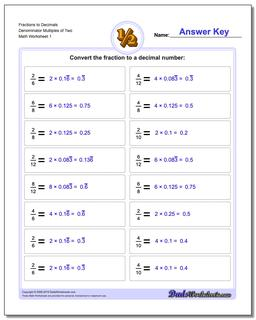# Math Worksheets: Fractions as Decimals: Fractions as Decimals: Fractions to Decimals Denominator Multiples of Two## Fractions to Decimals Denominator Multiples of Two

PropertyValue
DescriptionFractions to Decimals Denominator Multiples of Two: Drills for converting common fractions into decimal equivalents.
Resource TypeWorksheet